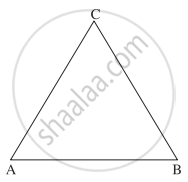# If two sides of a triangle are hat"i" + 2hat"j" and hat"i" + hat"k", find the length of the third side. - Mathematics and Statistics

Sum

If two sides of a triangle are hat"i" + 2hat"j" and hat"i" + hat"k", find the length of the third side.

#### SolutionLet ABC be a triangle with bar"AB" = hat"i" + 2hat"j", bar"BC" = hat"i" + hat"k".

By triangle law of vectors

bar"AC" = bar"AB" + bar"BC"

= (hat"i" + 2hat"j") + (hat"i" + hat"k")

= 2hat"i" + 2hat"j" + hat"k"

∴ l(AC) = |bar"AC"| = sqrt(2^2 + 2^2 + 1^2) = sqrt9 = 3 units

Hence, the length of third side is 3 units.

Concept: Vectors and Their Types
Is there an error in this question or solution?